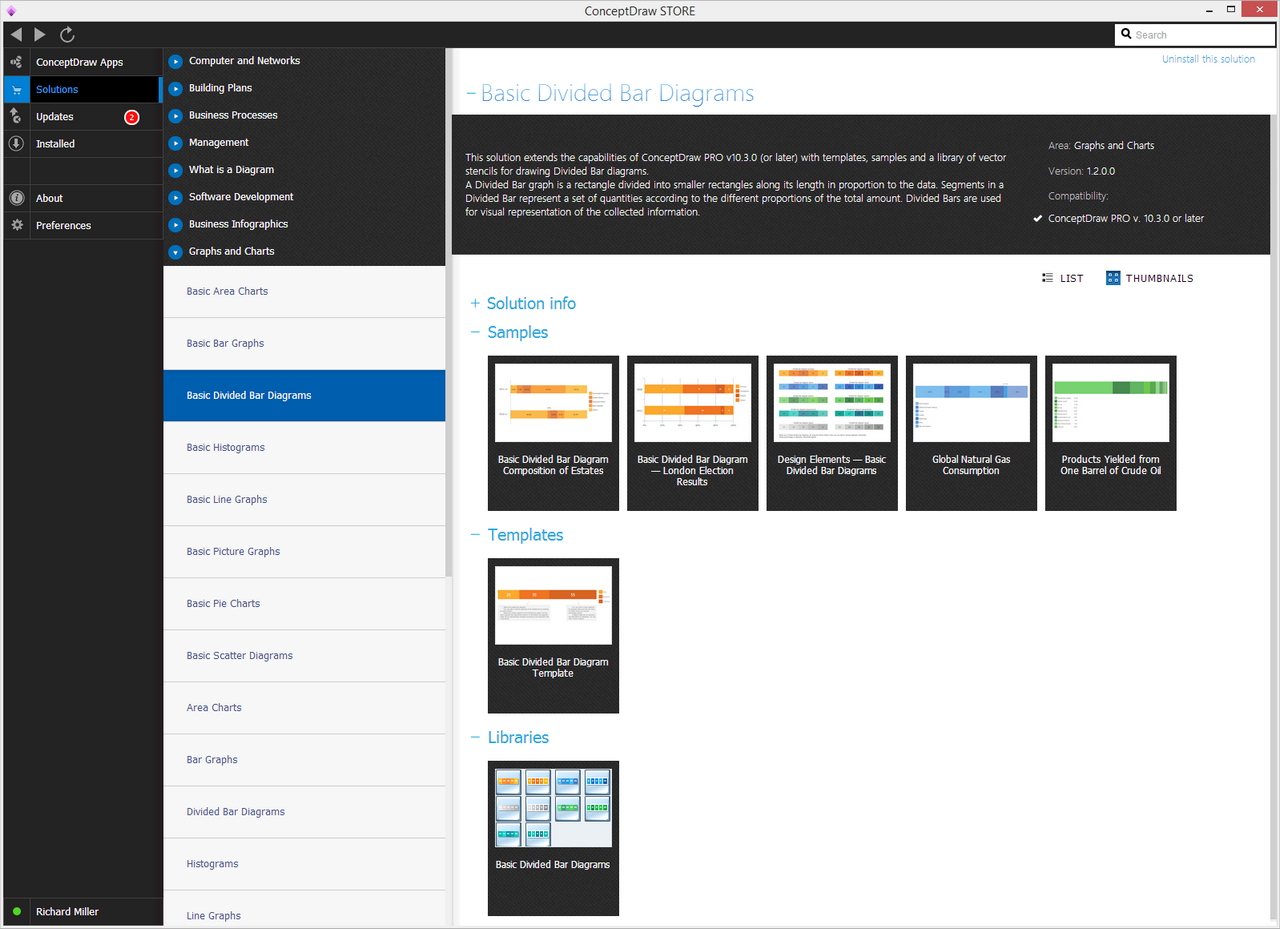This site uses cookies. By continuing to browse the ConceptDraw site you are agreeing to our Use of Site Cookies.# Basic Divided Bar Diagrams

This solution extends the capabilities of ConceptDraw DIAGRAM with templates, samples and a library of vector stencils for drawing Divided Bar diagrams.

A Divided Bar graph is a rectangle divided into smaller rectangles along its length in proportion to the data. Segments in a Divided Bar represent a set of quantities according to the different proportions of the total amount. Divided Bars are used for visual representation of the collected information.

There is 1 stencil library containing 10 vector objects in the Basic Divided Bar Diagrams solution.

## Design Elements — Basic Divided Bar Diagrams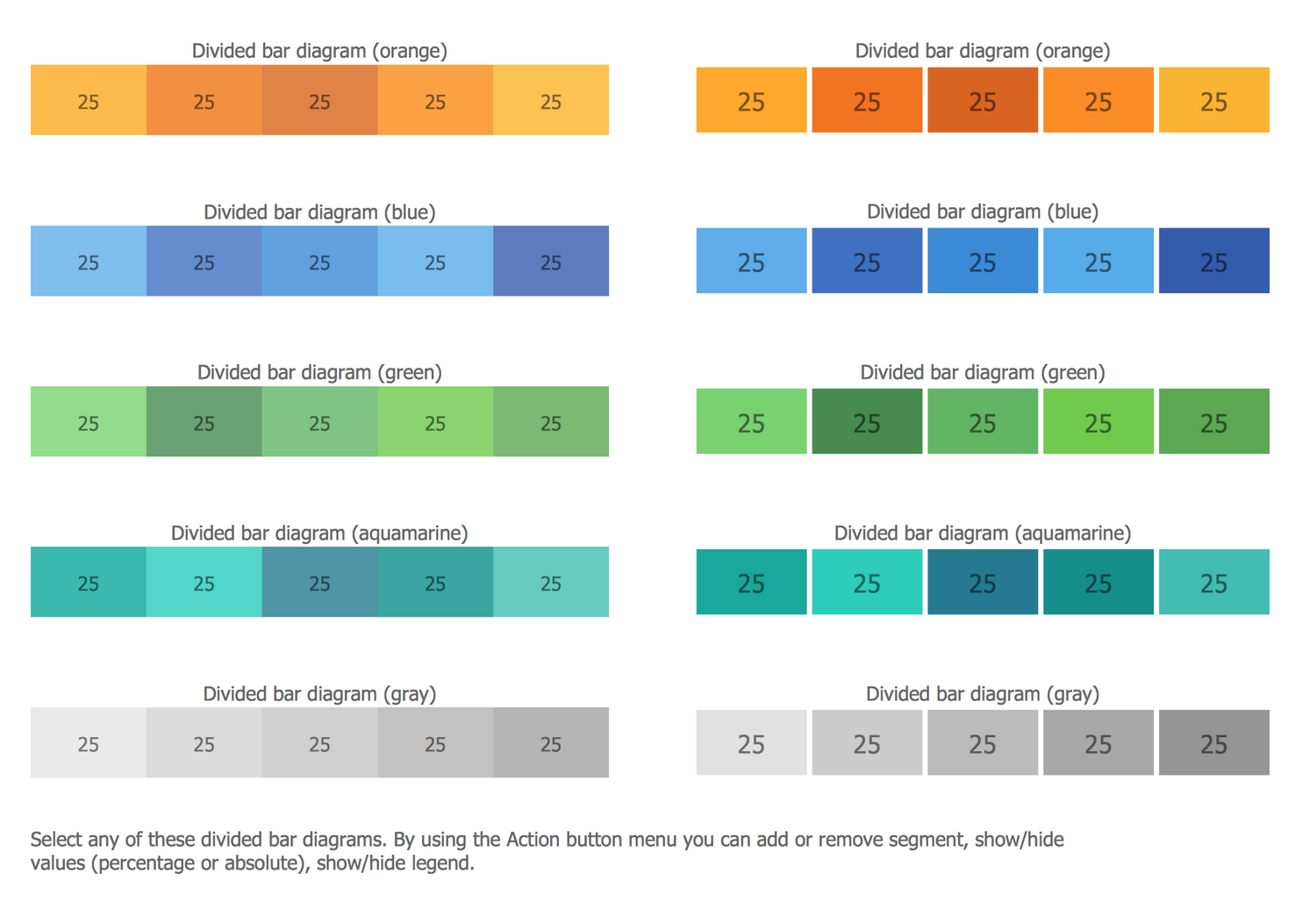## Examples

There are a few samples that you see on this page which were created in the ConceptDraw DIAGRAM application by using the Basic Divided Bar Diagrams solution. Some of the solution's capabilities as well as the professional results which you can achieve are all demonstrated here on this page.

All source documents are vector graphic documents which are always available for modifying, reviewing and/or converting to many different formats, such as MS PowerPoint, PDF file, MS Visio, and many other graphic ones from the ConceptDraw Solution Park or ConceptDraw STORE. The Basic Divided Bar Diagrams solution is available to all ConceptDraw DIAGRAM users to get installed and used while working in the ConceptDraw DIAGRAM diagramming and drawing software.

## Example 1: Basic Divided Bar Diagram Composition of Estates

This example was created in ConceptDraw DIAGRAM using the Basic Divided Bar Diagrams Library from the Basic Divided Bar Diagrams Solution. An experienced user spent 10 minutes creating this sample.

This example shows a combination of two divided bar diagrams. Looking at this diagram, you need just a few seconds to understand that the composition of estates has significantly changed. You can then pay attention to each bar to analyze and compare categories, individually.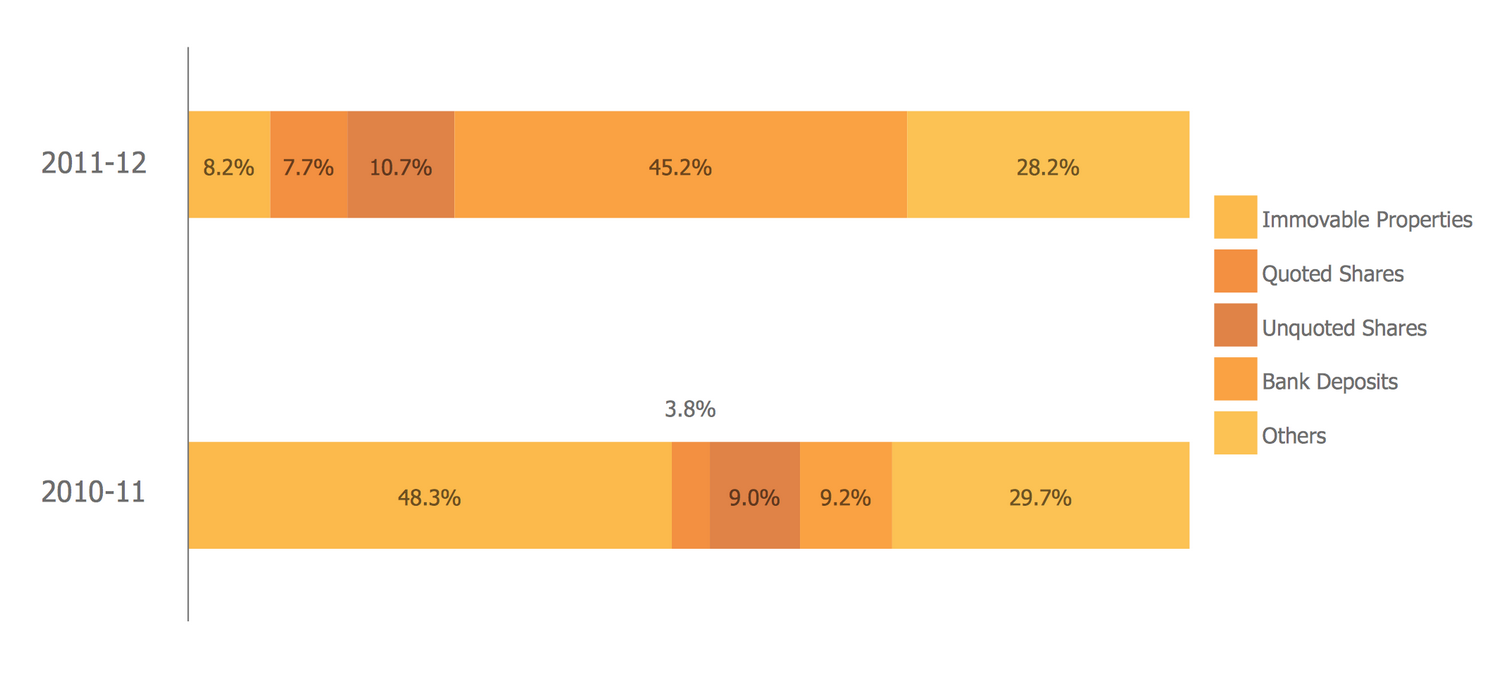## Example 2: Global Natural Gas Consumption

This example was created in ConceptDraw DIAGRAM using the Basic Divided Bar Diagrams Library from the Basic Divided Bar Diagrams Solution. An experienced user spent 5 minutes creating this sample.

Using a divided bar graph to compare data, you have many tools at your disposal to focus attention on different aspects of the comparison. One such tool is the order in which you put categories. You can use geographical, alphabetical or any other aspect of your data to highlights the main ideas of the comparison.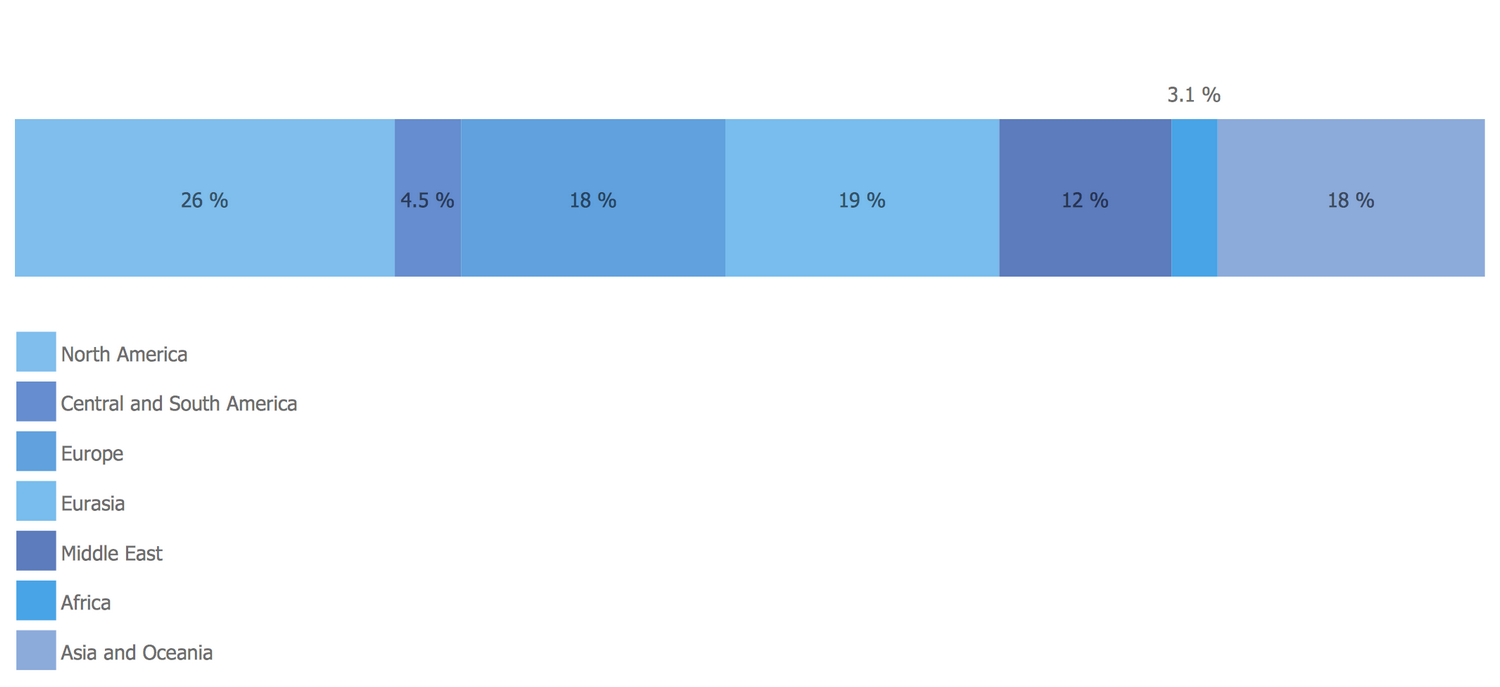## Example 3: Basic Divided Bar Diagram — London Election Results

This example was created in ConceptDraw DIAGRAM using the Basic Divided Bar Diagrams Library from the Basic Divided Bar Diagrams Solution. An experienced user spent 5 minutes creating this sample.

This example demonstrates using a combination of divided bar diagrams for comparing statistics. Adding axes makes this comparison easier to read.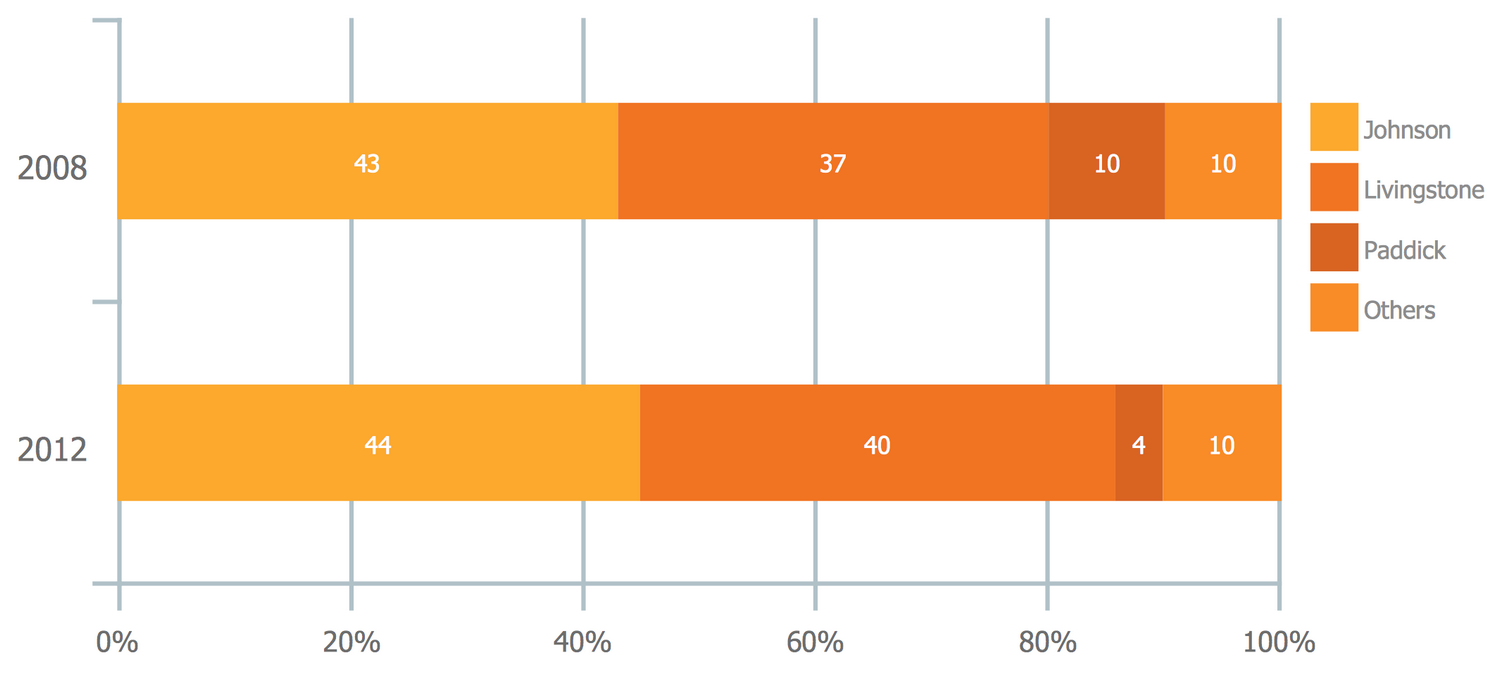## Example 4: Products Yielded from One Barrel of Crude Oil

This example was created in ConceptDraw DIAGRAM using the Basic Divided Bar Diagrams Library from the Basic Divided Bar Diagrams Solution. An experienced user spent 10 minutes creating this sample.

Using balanced color palettes makes divided bar diagrams stylish and attractive for modern presentations and infographics. We recommend keeping data as balanced as these color palates, using a limited number of categories. This allows you to keep your divided bar diagram as easy to read as it is pleasant to see.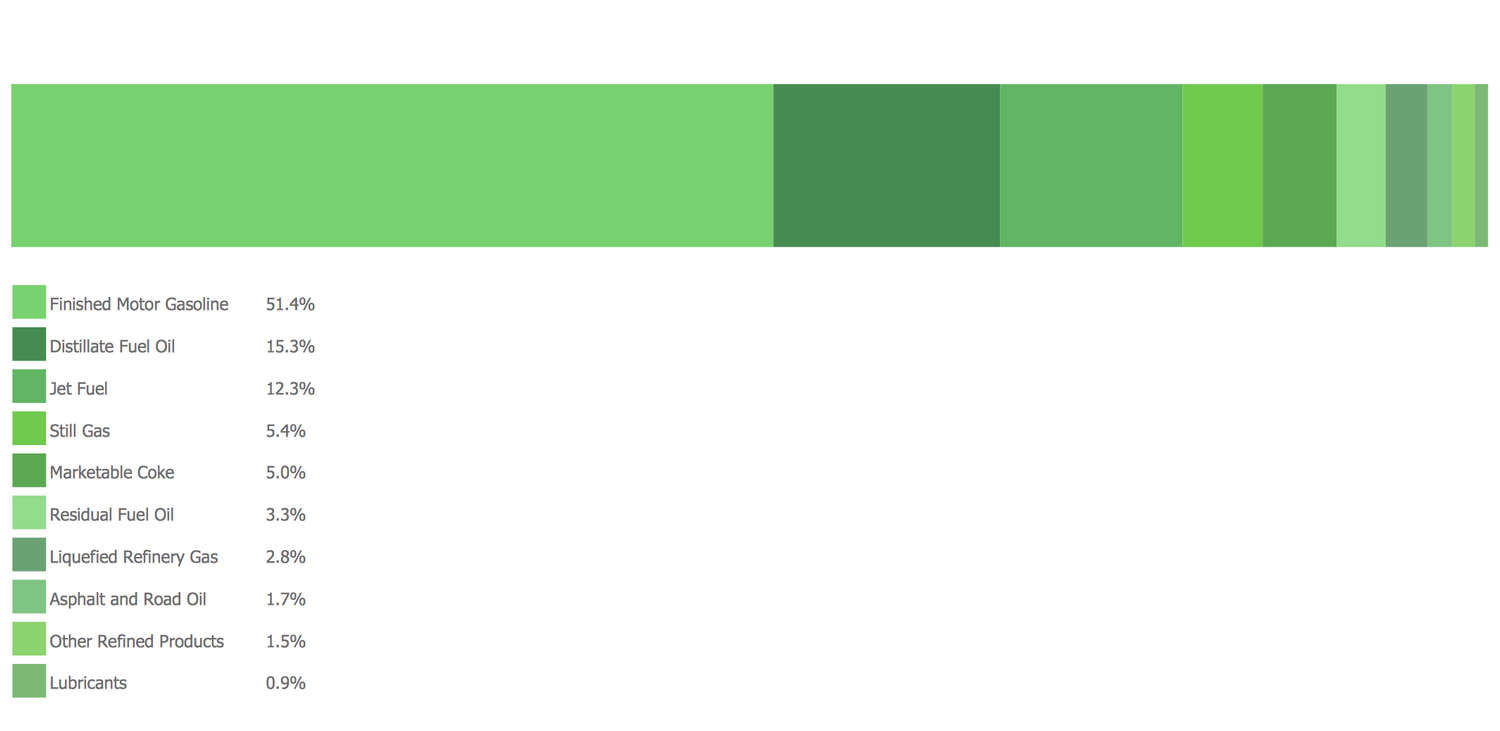## Inside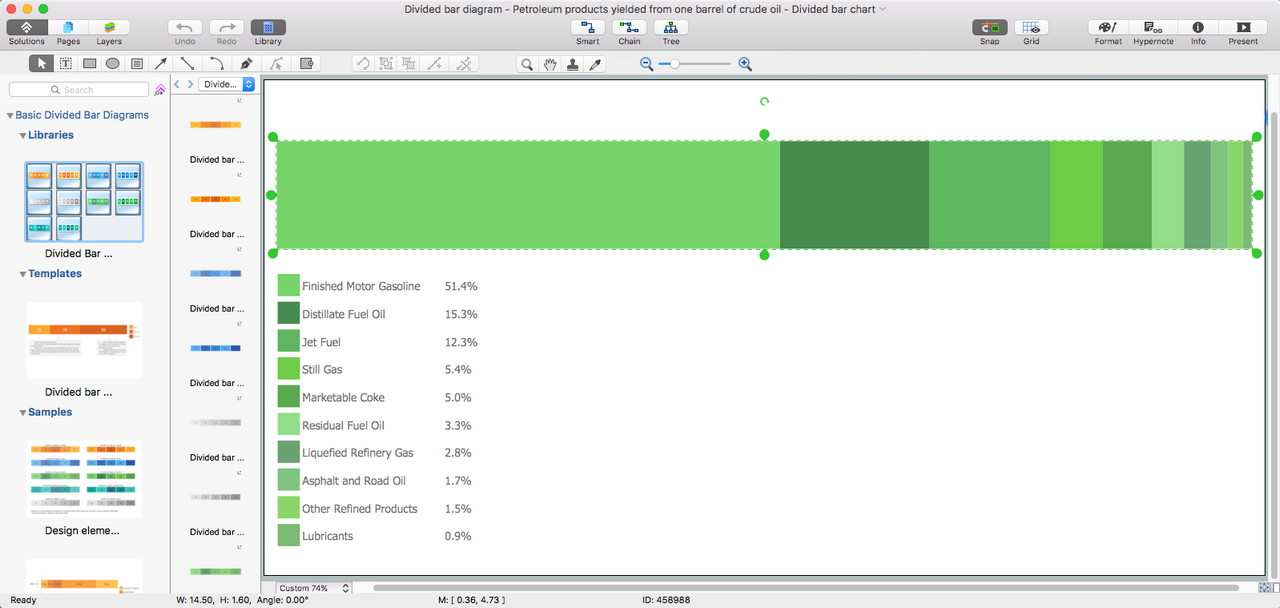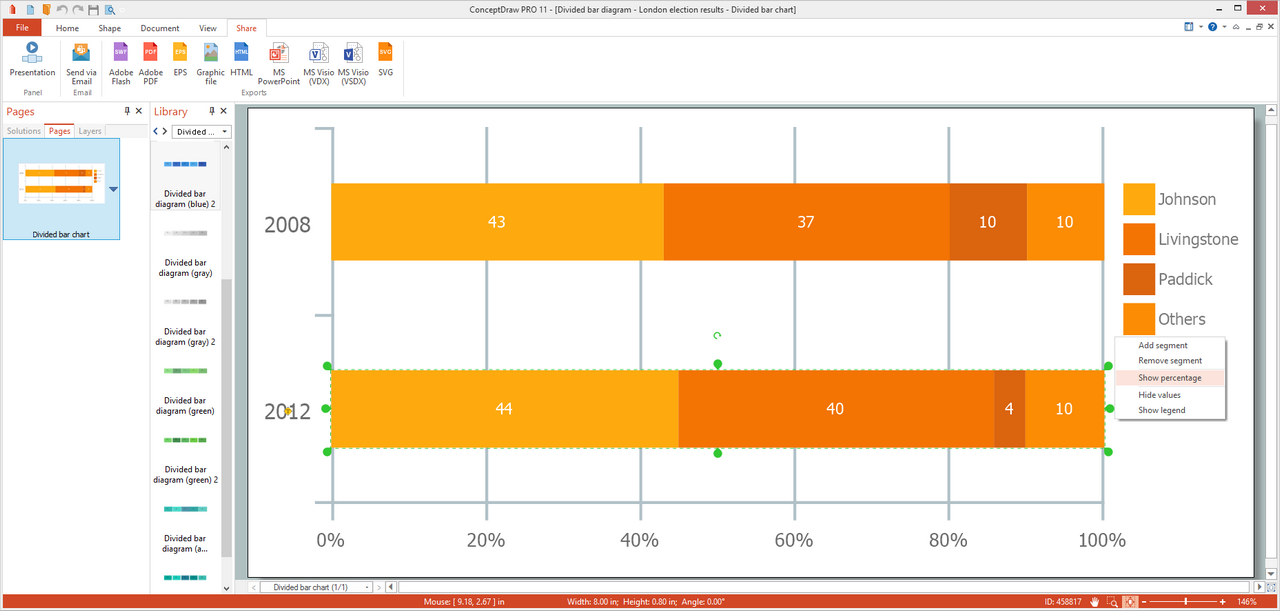## What I Need to Get Started

Both ConceptDraw DIAGRAM diagramming and drawing software and the Basic Divided Bar Diagrams solution can help creating the numerical data you need. The Basic Divided Bar Diagrams solution can be found in the Graphs and Charts area of ConceptDraw STORE application that can be downloaded from this site. Make sure that both ConceptDraw DIAGRAM and ConceptDraw STORE applications are installed on your computer before you get started.

#### How to install

After ConceptDraw STORE and ConceptDraw DIAGRAM are downloaded and installed, you can install the Basic Divided Bar Diagrams solution from the ConceptDraw STORE.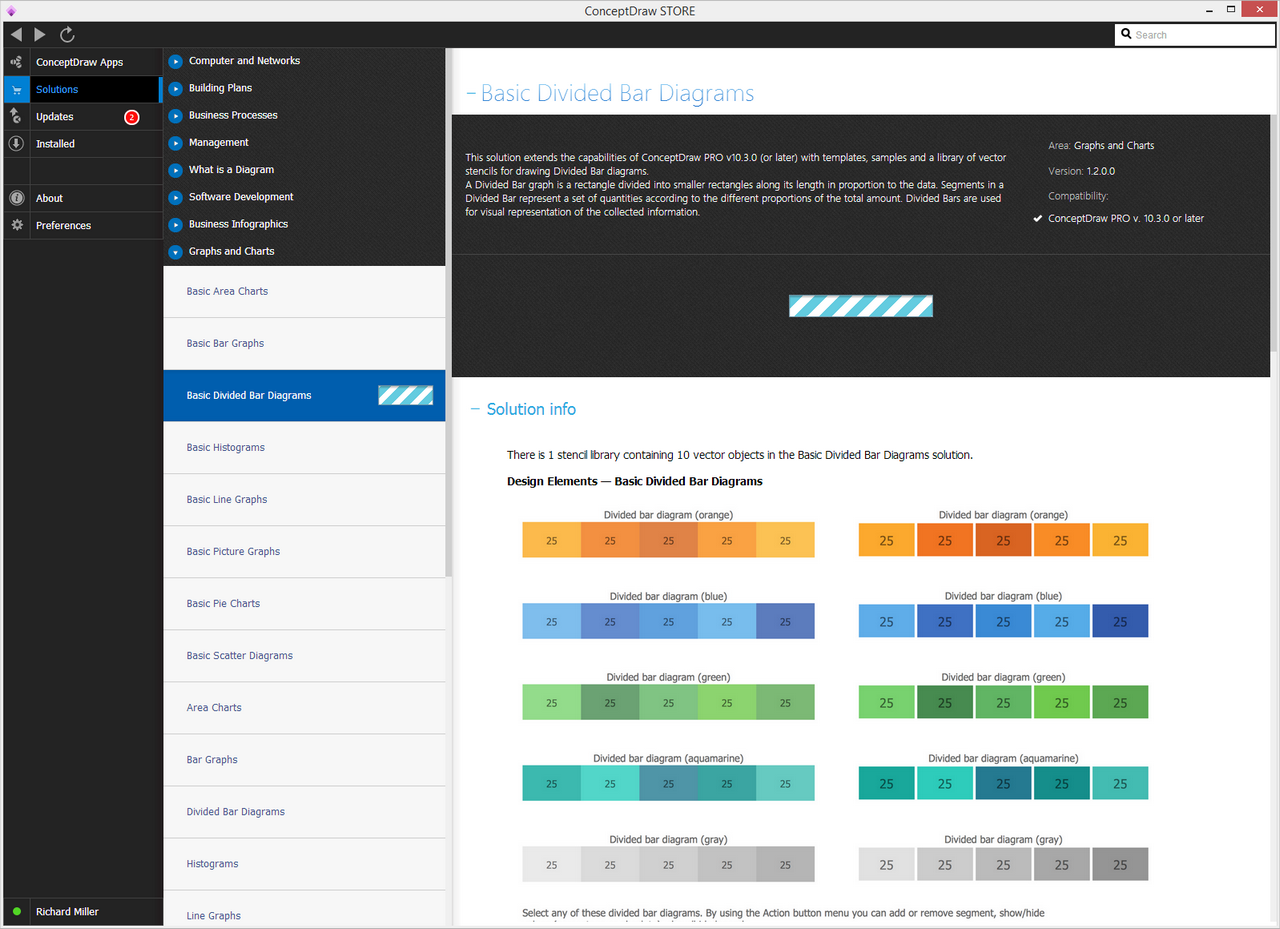#### Start Using

To make sure that you are doing it all right, use the pre-designed symbols from the stencil libraries from the solution to make your drawings look smart and professional. Also, the pre-made examples from this solution can be used as drafts so your own drawings can be based on them. Using the samples, you can always change their structures, colors and data.